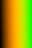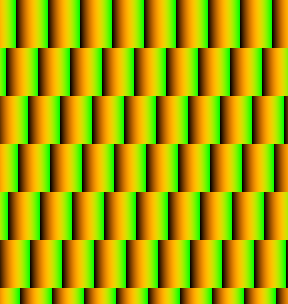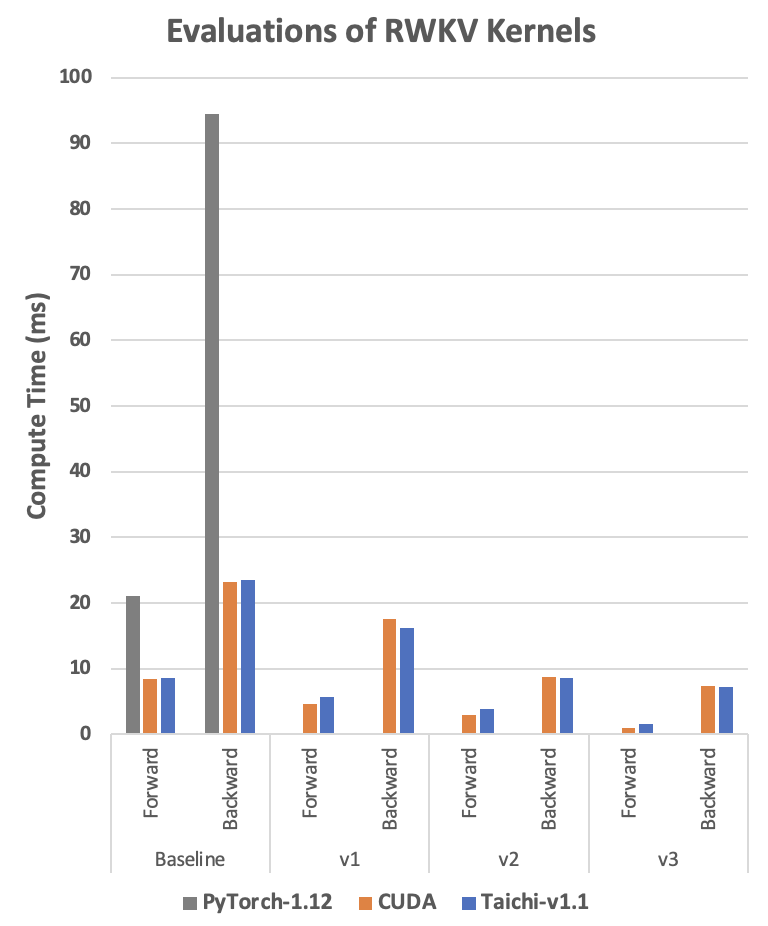Version: v1.6.0

# Accelerate PyTorch with Taichi

Taichi and Torch serve different application scenarios but can complement each other.

• Taichi provides finer control over parallelization and enables more 'granular' (element-level) operations, giving its users much more flexibilities.
• Torch abstracts such details into Tensor-level operations like LEGO bricks, enabling its users to focus on building ML (Machine Learning) models.

This document uses two examples to explain how to use Taichi kernel to implement data preprocessing operators and custom high-performance ML operators.

## Data preprocessing

This section uses padding as an example to show you how Taichi can complement PyTorch in data preprocessing.

Padding is a commonly-used data preprocessing technique in machine learning. For example, padding can prevent convolution operations from changing the size of the input image. However, no PyTorch operators are designed specifically for padding in a specific customized pattern. Previously, you have two options to work around this:

• Using Python or PyTorch to iterate over matrix elements.
• Writing a C++/CUDA operator and connecting it to PyTorch via Python's custom operator extension.

The former has very poor efficiency and could become a drain of the neural network training performance; the latter requires large amount of domain-specific knowledge about the underlying hardware architectures and it could take a long while to get started.

Now, you can use Taichi to pad a brick wall of a specific customized pattern in a much more efficient way.

The following sections compare PyTorch's implementation of this workflow with Taichi's implementation:

1. Create a 'brick' and fill it with changing colors.2. Repeat the bricks horizontally with a fixed offset to form a staggered layout.The following code implements a PyTorch kernel `torch_pad()` for padding. To improve efficiency, the kernel turns the padding process into a series of native PyTorch matrix operations. But such matrix operations are usually unintuitive and require so many intermediate results to be stored in the GPU memory that old GPUs with less RAM cannot even afford them.

``def torch_pad(arr, tile, y):    # image_pixel_to_coord    arr[:, :, 0] = image_height - 1 + ph - arr[:, :, 0]    arr[:, :, 1] -= pw    arr1 = torch.flip(arr, (2, ))    # map_coord    v = torch.floor(arr1[:, :, 1] / tile_height).to(torch.int)    u = torch.floor((arr1[:, :, 0] - v * shift_y) / tile_width).to(torch.int)    uu = torch.stack((u, u), axis=2)    vv = torch.stack((v, v), axis=2)    arr2 = arr1 - uu * shift_x - vv * shift_y    # coord_to_tile_pixel    arr2[:, :, 1] = tile_height - 1 - arr2[:, :, 1]    table = torch.flip(arr2, (2, ))    table = table.view(-1, 2).to(torch.float)    inds = table.mv(y)    gathered = torch.index_select(tile.view(-1), 0, inds.to(torch.long))    return gatheredwith Timer():    gathered = torch_pad(coords, tile, y)    torch.cuda.synchronize(device=device)``

The following code implements a Taichi kernel `ti_pad()` for padding. The kernel iterates over the pixels in the output image, works out each pixel's corresponding position in the input 'brick', and fills the pixel with the RGB color in that position.

Taichi automatically runs the top-level for-loops in parallel, and matrix operations written in Taichi are much more readable. Moreover, as you can tell from the following code, `ti_pad()` takes in the PyTorch tensors directly so that it can reuse the memory allocated by PyTorch and would not cause extra overhead from the data transfer between the two frameworks.

``@ti.kerneldef ti_pad(image_pixels: ti.types.ndarray(), tile: ti.types.ndarray()):    for row, col in ti.ndrange(image_height, image_width):        # image_pixel_to_coord        x1, y1 = ti.math.ivec2(col - pw, image_height - 1 - row + ph)        # map_coord        v: ti.i32 = ti.floor(y1 / tile_height)        u: ti.i32 = ti.floor((x1 - v * shift_y) / tile_width)        x2, y2 = ti.math.ivec2(x1 - u * shift_x - v * shift_y,                 y1 - u * shift_x - v * shift_y)        # coord_to_tile_pixel        x, y = ti.math.ivec2(tile_height - 1 - y2, x2)        image_pixels[row, col] = tile[x, y]with Timer():    ti_pad(image_pixels, tile）    ti.sync()``

### Performance comparison

As the following table shows, the PyTorch kernel takes 30.392 ms to complete padding; the Taichi kernel takes 0.267 ms only. Taichi outruns PyTorch by more than 100x (30.392/0.267).

`torch_pad()` launches 58 CUDA kernels, whilst Taichi compiles all computation into one CUDA kernel. The fewer the CUDA kernels, the less GPU launch overhead is incurred. Moreover, the Taichi kernel manages to save a lot more redundant memory operations than the PyTorch kernel. The GPU launch overhead and the redundant memory operations are the potential source for optimization and acceleration.

Kernel functionAverage time (ms)CUDA kernels launched (number)
`torch_pad()`30.39258
`ti_pad()`0.2671
• GPU: RTX3090
• PyTorch version: v1.12.1; Taichi version: v1.1.0
• The actual acceleration rate may vary depending on your implementation and GPU setup.

## Customize ML operators

Researchers in machine learning usually spend a lot of time designing model architectures. Because they cannot find decent support for their newly-designed or customized operators from PyTorch, they have to spend time studying CUDA for fine tuning and to improve efficiency. But writing in CUDA is hard, tuning CUDA code is even harder, and accelerating model iteration with CUDA is difficult.

This repo introduces an example of customizing an ML operator in CUDA. The author developed an RWKV language model using sort of a one-dimensional depthwise convolution custom operator. The model does not involve much computation but still runs slow because PyTorch does not have native support for it. So, the author customized the operator in CUDA using a set of optimization techniques, such as loop fusion and Shared Memory, and achieved a performance 20x better than he did with PyTorch.

Referring to the CUDA code3, we customized a Taichi depthwise convolution operator4 in the RWKV model using the same optimization techniques.

The function of the depth wise convolution operator:

1. Iterates over two input Tensors `w` and `k`,
2. Adds up the product of the respective elements in `w` and `k` into `s`,
3. Saves `s` to an output Tensor `out`.

The following subsections take the Baseline implementations as an example to show you how to implement a depthwise convolution operator with Python, PyTorch, CUDA, and Taichi, and how they compare to each other. With Taichi, you can accelerate your ML model development with ease and get rid of the tedious low-level parallel programming.

PythonExcellentThe slowest
PyTorchPoorSlow
CUDAPoorFast
TaichiExcellentComparable to that of CUDA or even better

### Implement a depthwise convolution operator with Python

The Python reference code is straightforward and easy to understand, but it runs so slow that the result can hardly make itself into the diagram below.

``def run_formula_very_slow(w, k, B, C, T, eps):    out = torch.empty((B, C, T), device='cpu')    for b in range(B):        for c in range(C):            for t in range(T):                s = eps                for u in range(t-T+1, t+1):                    s += w[c][(T-1)-(t-u)] * k[b][c][u+T-1]                out[b][c][t] = s    return out``

### Implement a depthwise convolution operator with PyTorch

It is very challenging to translate the Python reference code above to this code line. To come up with this, you have to know very well the underlying logic of these PyTorch operators.

``out = eps + F.conv1d(nn.ZeroPad2d((T-1, 0, 0, 0))(k), w.unsqueeze(1), groups=C)``

### Implement a depthwise convolution operator with CUDA

The CUDA reference code has much poorer readability: The outmost loop is implicitly defined by thread parallelism. The index calculation is complicated, and each element's position in the matrix is not clear at a glance. Besides, it could be rather error-prone to implement more sophisticated algorithms with CUDA.

``__global__ void kernel_forward(const float* w, const float* k, float* x,                               const float eps, const int B, const int C, const int T){    const int i = blockIdx.y;    const int t = threadIdx.x;    float s = eps;    const float* www = w + (i % C) * T + (T - 1) - t;    const float* kk = k + i * T;    for (int u = 0; u <= t; u++){        s += www[u] * kk[u];    }    x[i * T + t] = s;}``

Further, you need a proper compile environment to run your CUDA code! If you have precompiled your CUDA code into a dynamic link library, then you also need to spend time working hard on trivial matters such as environment settings and Python API encapsulation.

### Implement a depthwise convolution operator with Taichi

The Taichi reference code is almost identical to its Python counterpart. And a good advantage that Taichi has over CUDA is that, without worrying about low-level details like parallelization and pointer offsets, one can easily use Taichi to achieve comparable performance.

``@ti.kerneldef taichi_forward_v0(        out: ti.types.ndarray(ndim=3),        w: ti.types.ndarray(ndim=3),        k: ti.types.ndarray(ndim=3),        eps: ti.f32):    for b, c, t in out:        s = eps        for u in range(t-T+1, t+1):            s += w[c, 0, (T-1)-(t-u)] * k[b, c, u+T-1]        out[b, c, t] = s``

### Performance comparison

The following diagram shows that Taichi always shows a performance that is comparable to its CUDA counterpart or even better under certain circumstances.• The RWKV compute time in the diagram is in milliseconds. The less the compute time, the better the performance is.
• 'Baseline': The reference code is a faithful implementation of the algorithm without any modification.
• v1 to v3: The three different optimized implementations.

## Recap

PyTorch is efficient in handling a large proportion of computation tasks in machine learning. Still, there are niches and needs that it falls short of addressing, such as native support for many operators and unsatisfactory runtime performance.

As a high-performance programming language embedded in Python, Taichi features: